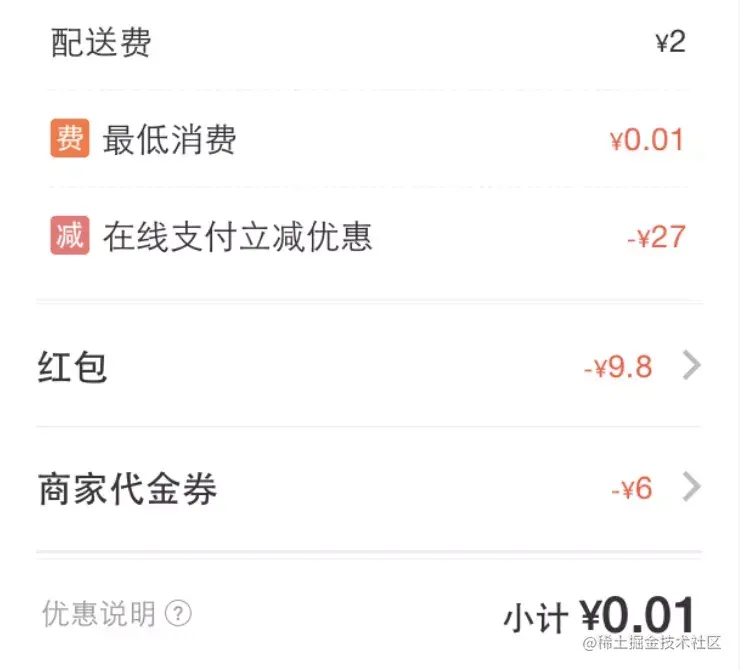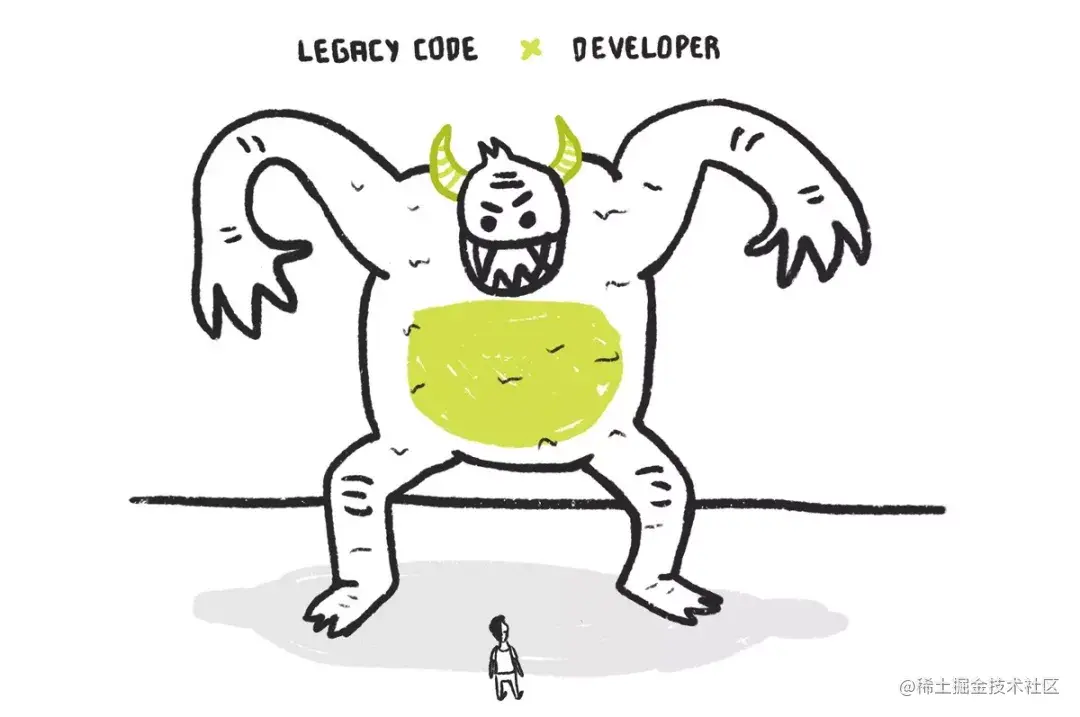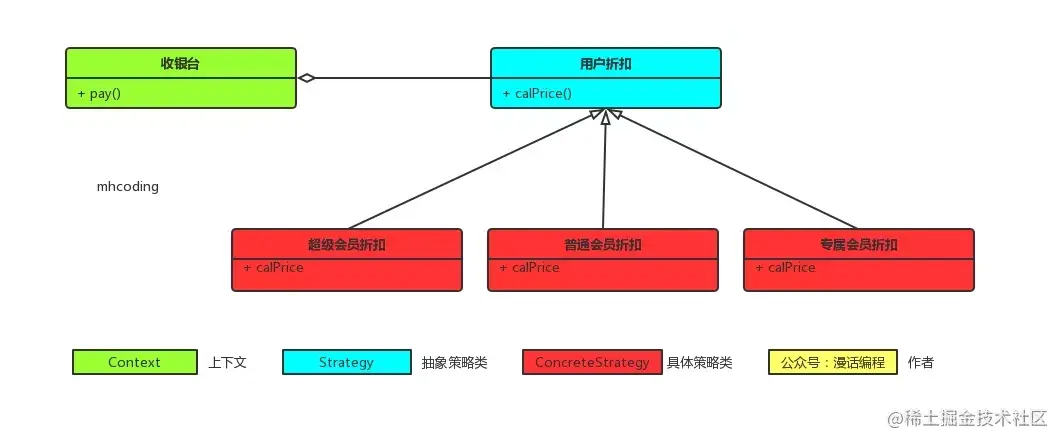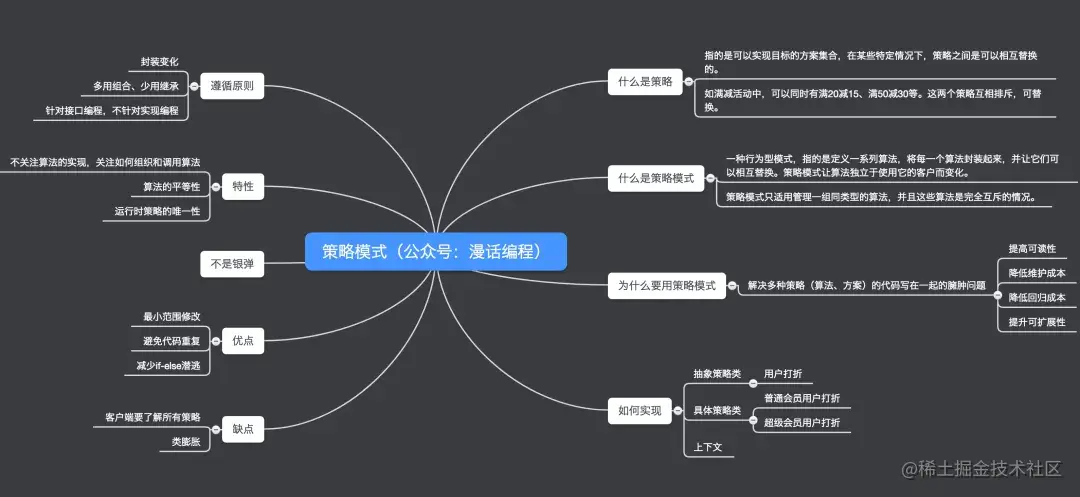# 漫话：如何给女朋友解释什么是策略模式？``````public BigDecimal calPrice(BigDecimal orderPrice, String buyerType) {

return orderPrice.multiply(new BigDecimal(0.8));
}

return orderPrice.multiply(new BigDecimal(0.9));
}

return orderPrice;
}``````public BigDecimal calPrice(BigDecimal orderPrice, String buyerType) {

return orderPrice.multiply(new BigDecimal(0.7));
}

return orderPrice.multiply(new BigDecimal(0.8));
}

return orderPrice.multiply(new BigDecimal(0.9));
}

return orderPrice;
}

``````public BigDecimal calPrice(BigDecimal orderPrice, String buyerType) {

if (orderPrice.compareTo(new BigDecimal(30)) > 0) {
return orderPrice.multiply(new BigDecimal(0.7));
}
}

return orderPrice.multiply(new BigDecimal(0.8));
}

return orderPrice.multiply(new BigDecimal(0.9));
}

return orderPrice;
}

``````public BigDecimal calPrice(BigDecimal orderPrice, String buyerType) {

if (orderPrice.compareTo(new BigDecimal(30)) > 0) {
return orderPrice.multiply(new BigDecimal(0.7));
}
}

return orderPrice.multiply(new BigDecimal(0.8));
}

int  superVipExpiredDays  = getSuperVipExpiredDays();
if(superVipExpiredDays < 7 && superVipLeadDiscountTimes =0){
return orderPrice.multiply(new BigDecimal(0.8));
}
return orderPrice.multiply(new BigDecimal(0.9));
}

return orderPrice;
}``````public interface Buyer {

/**
* 计算应付价格
*/
public BigDecimal calPrice(BigDecimal orderPrice);
}

``````/**
* 专属会员
*/

@Override
public BigDecimal calPrice(BigDecimal orderPrice) {
if (orderPrice.compareTo(new BigDecimal(30)) > 0) {
return orderPrice.multiply(new BigDecimal(0.7));
}
}
}

/**
* 超级会员
*/

@Override
public BigDecimal calPrice(BigDecimal orderPrice) {
return orderPrice.multiply(new BigDecimal(0.8));
}
}

/**
* 普通会员
*/

@Override
public BigDecimal calPrice(BigDecimal orderPrice) {
int  superVipExpiredDays  = getSuperVipExpiredDays();
if(superVipExpiredDays < 7 && superVipLeadDiscountTimes =0){

return orderPrice.multiply(new BigDecimal(0.8));
}
return orderPrice.multiply(new BigDecimal(0.9));
}
}

``````public class Cashier {

/**
* 会员,策略对象
*/

}

public BigDecimal quote(BigDecimal orderPrice) {
}
}

``````public class Test {

public static void main(String[] args) {

//选择并创建需要使用的策略对象
//创建上下文
Cashier cashier = new Cashier(strategy);
//计算价格
BigDecimal quote = cashier.quote(300);
System.out.println("普通会员商品的最终价格为：" + quote.doubleValue());

cashier = new Cashier(strategy);
quote = cashier.quote(300);
System.out.println("超级会员商品的最终价格为：" + quote.doubleValue());
}
}

``````//普通会员商品的最终价格为：270.0
//超级会员商品的最终价格为：240.0• 策略模式的关注点不是如何实现算法，而是如何组织、调用这些算法，从而让程序结构更灵活，具有更好的维护性和扩展性。

• 策略模式中各个策略算法是平等的。对于一系列具体的策略算法，大家的地位是完全一样的，正因为这个平等性，才能实现算法之间可以相互替换。所有的策略算法在实现上也是相互独立的，相互之间是没有依赖的。所以可以这样描述这一系列策略算法：策略算法是相同行为的不同实现。

• 运行期间，策略模式在每一个时刻只能使用一个具体的策略实现对象，虽然可以动态地在不同的策略实现中切换，但是同时只能使用一个。• 策略模式提供了对“开闭原则”的完美支持，用户可以在不修改原有系统的基础上选择算法或行为，也可以灵活地增加新的算法或行为。

• 策略模式提供了管理相关的算法族的办法。策略类的等级结构定义了一个算法或行为族。恰当使用继承可以把公共的代码移到父类里面，从而避免代码重复。

• 使用策略模式可以避免使用多重条件(if-else)语句。多重条件语句不易维护，它把采取哪一种算法或采取哪一种行为的逻辑与算法或行为的逻辑混合在一起，统统列在一个多重条件语句里面，比使用继承的办法还要原始和落后。

• 客户端必须知道所有的策略类，并自行决定使用哪一个策略类。这就意味着客户端必须理解这些算法的区别，以便适时选择恰当的算法类。这种策略类的创建及选择其实也可以通过工厂模式来辅助进行。

• 由于策略模式把每个具体的策略实现都单独封装成为类，如果备选的策略很多的话，那么对象的数目就会很可观。可以通过使用享元模式在一定程度上减少对象的数量。